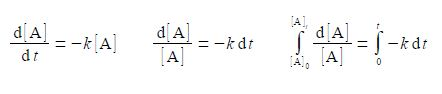# Explain Radioactive Decay with Integration

The decay of a radioactive element A → P has a rate constant k. Radioactive decay is a first-order chemical reaction with [A] and t being the variables of the equation.

d[A] / dt = – k [A]

The rate of reaction is equal to the rate of loss of [A] with time t which is equal to the product of the rate constant k and the concentration [A]. The negative sign is to give a positive rate constant for a reaction which involves the loss of the reactant. In order to obtain an equation which will give us the concentration of A at any time we need to integrate this equation from t = 0 when [A] = [A]0) to a general time t = t when [A] = [A]t. Carry out this definite integration for this first-order chemical reaction.

We are going to introduce the “separation of variables” method with this question and its solution.

d[A] / dt = – k [A]

(1) Treat d[A] and dt as two separate variables which follow the normal rules of algebra (you cannot separate d from t as the operator d has to operate on something, this applies to any differential).

(2) Rearrange the equation to bring everything that involves [A] to the left-hand side and everything that involves tor are constants to the right-hand side of the equation.

(3) You can now integrate both sides of the equation separately, using the two appropriate sets of integration limits.(4) Take any constants and negative signs outside of the integral for clarity, i.e. the rate constant k is independent of time and is constant.

(5) The left and right-hand sides of the equation are both standard integrals, the left-hand side making use of the following standard integral(6) This gives four alternative ways of writing the final definite integral and which one you use to solve a problem will depend upon the circumstances.

ln [A]t – ln [A]0 = -kt

ln {[A]t / ln [A]0} = – kt

{[A]t / ln [A]0} = e– kt

[A]t = [A]0 e– kt